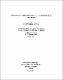## Inclusive measurements of the pp-&gt;pn[pi]S+ reaction at 420 and 500 MeVInclusive measurements of the pion differential cross sections and analyzing powers have been made for the reaction $pp\to pn\pi\sp+$ over the angular range 24$\sp\circ$ to 100$\sp\circ,$ using the SASP spectrometer at TRIUMF. A 1 cm thick liquid hydrogen target was used, with an identical empty cell for background determination. These studies included the $pp\to d\pi\sp+$ reaction for verification of the cross section normalization. Measurements extended to pion momenta as low as 80 MeV/c. Experimental cross sections are compared with a final state interaction model prediction and the contribution of the $\sigma\sb{11}$ term. The analyzing powers closely follow those of the $pp\to d\pi\sp+$ reaction at the same centre of mass momentum and energy.